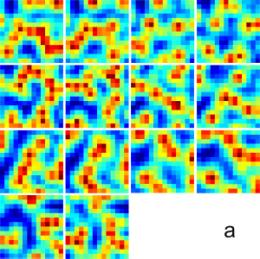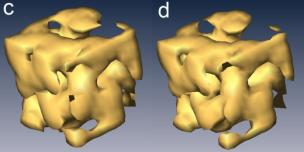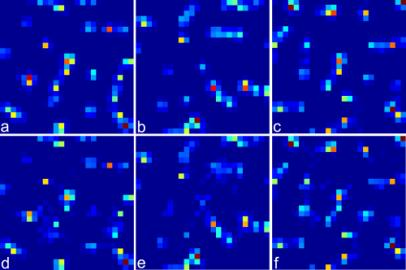Matlab Source Codes Used for Ankylographic Reconstructions

If any of the following codes are used in your publications and/or presentations, we request you cite our paper (i.e. ref. 1).

I). Numerical simulation on the ankylographic reconstruction of a 3D "UCLA" pattern with 7 x 7 x 7 voxels (posted on Jan. 20, 2011)Figure 1. Numerical simulation on ankylographic reconstruction of a 3D "UCLA" pattern from a spherical diffraction pattern of 1 voxel thick with a diffraction angle (2q) of 90 degree. The array size of the 3D pattern is 7 x 7 x7 voxels and oversampling degree (Od) is 1.14 . The upper panel shows the 1st, 3rd, 5th and 7th slices of the reconstructed image. The lower panel shows the corresponding slices of the 3D pattern, consisting four alphabet letters "U", "C", "L", and "A".

II). Numerical simulation on the ankylographic reconstruction of a continuous 3D object with 14 x 14 x 14 voxels (posted on Oct. 26, 2011)Figure 2. Numerical simulation on the ankylographic reconstruction of a continuous object with array size of 14 x 14 x 14 voxel. a, 14 slices of the 3D object with a minimum and a maximum voxel vale of 1.45 and 11.06, respectively. b, 14 reconstructed slices, which are in good agreement with the original ones. The reconstruction was computed from a simulated spherical different pattern of 1 voxel thick with a diffraction angle (2q) of 90 degree. The oversampling degree (Od) is 1.48  and the number of iteration is 106. c,d, Iso-surface renderings of the original and reconstructed object. The object is continuous and the holes in the images are due to a threshold value chosen for the display purpose.

If you are interested in reconstructing this continuous 3D object, please click here to download the Matlab code. In the reconstruction, we usually started with 100 random initial phase seeds and then chose the best one for the final reconstruction.

III). Numerical simulation on the ankylographic reconstruction of a sodium silicate glass structure with 25 x 25 x 25 voxels (posted on Oct. 26, 2011)Figure 3. Numerical simulation on the ankylographic reconstruction of a sodium silicate glass particle. The glass particle structure was generated by molecular dynamics simulations and consists of a total of 365 atoms with a resolution of 1.5 Angstrom (i.e. 0.75 Angstrom per pixel) and array size of 25 x 25 x 25 voxels. a-c, Three central slices of the glass structure along the XY, YZ and XZ planes. d-f, The corresponding reconstructed slices along the XY, YZ and XZ planes. The reconstruction was computed from a simulated spherical different pattern of 1 voxel thick with a diffraction angle (2q) of 90 degree. The oversampling degree (Od) is 1.50  and the number of iteration is 2x105.

If you are interested in reconstructing the simulated 3D sodium silicate glass particle, please click here to download the Matlab code. In the reconstruction, we usually started with 100 random initial phase seeds and then chose the best one for the final reconstruction.

References

1. K. S. Raines, S. Salha, R. L. Sandberg, H. Jiang, J. A. Rodriguez, B. P. Fahimian, H. C. Kapteyn, J. Du and J. Miao. Three-dimensional structure determination from a single view. Natrue 463, 214-217 (2010).

2. P. Thibault. Feasibility of 3D reconstruction from a single 2D diffraction measurement, arXiv:0909.1643v1 [physics.data-an] (2009).

3. J. Miao. Response to "Feasibility of 3D reconstruction from a single 2D diffraction measurement", arXiv:0909.3500v1 [physics.optics] (2009).

4.C.-C. Chen, H. Jiang, L. Rong, S. Salha, R. Xu, T. G. Mason and J. Miao. Three-dimensional imaging of a phase object from a single sample orientation using an optical laser. Phys. Rev. B 84, 224104 (2011).

5. M. D. Seaberg, D. E. Adams, E. L. Townsend, D. A. Raymondson, W. F. Schlotter, Y. Liu, C. S. Menoni, L. Rong, C.-C. Chen, J. Miao, H. C. Kapteyn and M. M. Murnane. Ultrahigh 22 nm resolution coherent diffractive imaging using a desktop 13 nm high harmonic source. Opt. Express 19, 22470-22479 (2011).